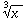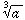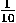# Appendix B

This appendix was copied with permission from Nick Strobel’s Astronomy Notes. Go to his site at www.astronomynotes.com for the updated and corrected version.

# Quick Mathematics Review

Here’s a quick run through of some basic mathematics: working with fractions and percentages, exponents, roots, powers of ten, working with really BIG or really small numbers—scientific notation and the metric system. I assume that the reader has had this stuff before, so the quick run through will be sufficient to jog the dormant memory.

This web page provides a review of the mathematical concepts you will apply in this course.

### Fractions and Percentages %

Fractions are a part of every-day life and they are also used in science. A fraction with the same numerator (number on top) as another but with a smaller denominator (number on bottom) is a larger number. For example 1/2 is bigger than 1/3 which is bigger than 1/4, etc. That is because if you divide 1 by 2, you get 0.5 but 1 divided by 3 is only 0.333 and 1 divided by 4 is only 0.25. The fraction 3/5 (=0.6) is bigger than 3/6 (=0.5) which is bigger than 3/7 (=0.4286).

Fractions are sometimes expressed as a percent. To express a fraction as a percentage, find the decimal form and multiply by 100. The percent symbol “%” simply means “divide by 100.” For example 1/2 = 0.5 = 0.5× 100% = 50%; 3/5 = 0.6 = 0.6× 100% = 60%. Some examples on converting percentages to fractions or decimals: 5.8% = 5.8/100 = 0.058; 0.02% = 0.02/100 = 0.0002; the Sun is 90% hydrogen means that 90 out of every 100 atoms in the Sun is hydrogen.

### “Times” and “Factor of”

Several homework questions ask you to find some quantity and find out how many “times” smaller or larger it is than something else, e.g., star A is ____times larger than star B. This means star A = a × star B, and you must find the number a. Another example: A gallon is equivalent to 4 quarts. This means that 1 gallon is 4 times bigger than 1 quart since 1 gallon = 4 × 1 quart, or (1 gallon)/(1 quart) = 4/1. This also means that 1 quart is 4 times smaller than 1 gallon since 1 quart = 1/4 × (1 gallon), or (1 quart)/(1 gallon) = 1/4. Notice when “times bigger” is used and when “times smaller” is used.

The use of the phrase “factor of” is very similar to the use of “times.” For example, 1 quart is a factor of 4 smaller than 1 gallon, or 1 gallon is a factor of 4 bigger than 1 quart.

### Exponents

A shorthand way to express a quantity multiplied by itself one or more time is to use a superscript number called an exponent. So

 a = a1 a × a = a2 (not 2 × a!) a × a × a = a3 (not 3 × a!) a × a × a × a = a4 a × a × a × a × a = a5

The quantity “a squared” means a2, “a cubed” means a3, and more generally, “a to the nth power” is an.

Some special rules apply when you divide or multiply numbers raised to some power. When you have an multiplied by am, the result is a raised to a power that is the sum of the exponents:

an × am = an+mWhen you have an divided by am, the result is araised to a power that is the difference of the exponents—the exponent on the bottom is subtracted from the exponent on the top:

(an) / (am) = an-m (not am-n!)When you have an raised to a power m, you multiply the exponents:

(an)m = an × m = anm (not an+m!)Negative exponents are used for reciprocals:

1 / a = a-1, 1 / (a2) = a-2, 1 / (a3) = a-3, 1 / (a4) = a-4, etc.Scientific calculators have a “yx” key or a “xy” that takes care of raising numbers to some exponent. Some fancy calculators have a “^” key that does the same thing. Some calculators have “x2” and “x3” keys to take care of those frequent squaring or cubing of numbers. Check your calculator’s manual or your instructor. The Basic Skills Computer Lab has some excellent software that can improve your skills with exponents. Try it out!

### Roots

The square root of a quantity is a number that when multiplied by itself, the product is the original quantity:

Sqrt[a] × Sqrt[a] = a. Some examples: Sqrt = 1 because 1 × 1 = 1; Sqrt = 2 because 2 × 2 = 4; Sqrt[38.44] = 6.2 because 6.2 × 6.2 = 38.44; Sqrt[25A2] = 5A because 5A × 5A = 25A2.

A square root of a number less than 1, gives a number larger than the number itself: Sqrt[0.01] = 0.1 because 0.1 × 0.1 = 0.01 and Sqrt[.36] = 0.6 because 0.6 × 0.6 = 0.36.

The cube root of a quantity is a number that when multiplied by itself two times, the product is the original quantity:

Cube-Root[a] × Cube-Root[a] × Cube-Root[a] = a.Scientific calculators have “Sqrt(x)” and sometimes “” keys to take care of the common square roots or cube roots. An expressionmeans the nth root of a. How can you use your calculator for something like that? You use the fact that the nth root of a is a raised to a fractional exponent of 1/n. So we have:= a1/n Sqrt[a] == a1/2 Cube-root[a] == a1/3

When you raise some nth root to some power m, you simply multiply the exponents as you did above for (an)m:

()m = (a1/n)m = a1/n × m = am/n. So (a6)1/2 = a6× (1/2) = a3 and (a1/2)6 = a(1/2)× 6 = a3. But if you have a1/n multiplied by am, you add the exponents since you are not raising a1/n to some power m: a1/2 × a6 = a(1/2) + 6 = a6 & 1/2 = a13/2.

### Powers of Ten

For numbers larger than 10, the power of 10 is a positive value and negative for numbers less than 1. For numbers between 0 and 10, the power is a positive fraction. In the examples that follow, notice what happens to the decimal point:

 100 = 1. = 1. with the decimal point moved 0 places 101 = 10. = 1. with the decimal point moved 1 place to the right 102 = 100. = 1. with the decimal point moved 2 places to the right 106 = 1000000. = 1. with the decimal point moved 6 places to the right and 10-1 = 0.1 = 1. with the decimal point moved 1 place to the left 10-2 = 0.01 = 1. with the decimal point moved 2 places to the left 10-6 = 0.000001 = 1. with the decimal point moved 6 places to the left.

The exponent of 10 tells you how many places to move the decimal point to the right for positive exponents or left for negative exponents. These rules come in especially handy for writing very large or very small numbers.

### Scientific Notation

Since you will be working with very large and very small numbers, use scientific notation to cut down on all of the zeroes you need to write. Proper scientific notation specifies a value as a number between 1 and 10 (called the mantissa below) multiplied by some power of ten, as in

mantissa × 10exponent.The power of ten tells you which way to move the decimal point and by how many places. As a quick review:

10 = 1 × 101, 253 = 2.53 × 100 = 2.53 × 102 and 15,000,000,000 = 1.5 × 1010 which you will sometimes see written as 15 × 109 even though this is not proper scientific notation. For small numbers we have:= 1 × 10-1,× 10-2 or about 0.395 × 10-2 = 3.95 × 10-3.

#### Multiplying and Dividing with Scientific Notation

When you multiply two values given in scientific notation, multiply the mantissa numbers and add the exponents in the power of ten. Then adjust the mantissa and exponent so that the mantissa is between 1 and 10 with the appropriate exponent in the power of ten. For example: (3×1010) × (6× 1023), you’d have 3×6 × 10(10+23) = 18. × 1033 = 1.8×1034.

When you divide two values given in scientific notation, divide the mantissa numbers and subtract the exponents in the power of ten. Then adjust the mantissa and exponent so that the mantissa is between 1 and 10 with the appropriate exponent in the power of ten. For example:× 1010-23 = 0.5 × 10-13 = 5 × 10-14.

Notice what happened to the decimal point and exponent in the examples. You subtract one from the exponent for every space you move the decimal to the right. You add one to the exponent for every space you move the decimal to the left.

#### Entering scientific notation on your scientific calculator

Please read this if you have not used a scientific calculator for a while!

Most scientific calculators work with scientific notation. Your calculator will have either an “EE” key or an “EXP” key. That is for entering scientific notation. To enter 253 (2.53 × 102), you would punch 2.53EE or EXP2. To enter 3.95 × 10-3, you would punch 3.95EE or EXP3[key]. Note that if the calculator displays “3.53 -14” (a space between the 3.53 and -14), it means 3.53 × 10-14 NOT 3.53-14! The value of 3.53-14 = 0.00000002144 = 2.144×10-8 which is vastly different than the number 3.53×10-14. Also if you have the number 4 × 103 and you enter 4×10EE or EXP3, the calculator will interpret that as 4 × 10 × 103 = 4 × 104 or ten times greater than the number you really want!

One other word of warning: the EE or EXP key is used only for scientific notation and NOT for raising some number to a power. To raise a number to some exponent use the “yx” or “xy” key depending on the calculator. For example, to raise 3 to the 4th power as in 34 enter 3yx or xy4. If you instead entered it using the EE or EXP key as in 3EE or EXP4, the calculator would interpret that as 3×104 which is much different than 34 = 81.

### Metric System

The metric system is a much more logical and straightforward system than the old English system still used in the United States. Every other nation in the world uses the metric system, so in today’s global economy, it is very advantageous to learn how to use the system. It is also the system used in science.

#### Prefixes

The metric system is based on the number 10, for example there are 10 millimeters in one centimeter, 1000 grams in one kilogram, 100 centimeters in one meter, 100 degrees between the freezing and boiling point of water, etc. Contrast this with the english system that has 12 inches to 1 foot, 16 ounces to 1 pound, 5,280 feet to one mile, 180 degrees Fahrenheit between the freezing and boiling point of water, … egad! All of the units in the metric system use a common set of prefixes:

prefix meaning example
pico 10-12 = 1/1012 1 picosecond = 10-12 second
nano 10-9 = 1/109 1 nanometer = 10-9 meter
micro 10-6 = 1/106 1 microgram = 10-6 gram
milli 10-3 = 1/1000 1 millikelvin = 10-3 kelvin
centi 10-2 = 1/100 1 centimeter = 1/100th meter
kilo 1000 1 kilogram = 1000 grams
mega 106 1 megasecond = 106 seconds
giga 109 1 gigakelvin = 109 kelvins
tera 1012 1 terameter = 1012 meters

#### Length

The base unit of length in the metric system is the meter. It is a little over 1 yard long, more precisely 39.37 inches long. Here are some other conversions:

 1 meter (m) = 39.37 inches = 1.094 yards (about one big step) 1 kilometer (km) = 1000 meters 0.62137 mile 1 centimeter (cm) = 1/100th meter 0.3937 inch (1/2.54 inch, about width of pinky))

#### Mass

The base unit of mass is the gram. Mass is the amount of material in something and is different than weight. See the gravity chapter for an explanation of mass, weight and the difference between the two. There are 28.3495 grams in one ounce. A paperclip has a mass of roughly one gram. Here are some other conversions:

 1 gram (g) = 0.0353 ounce produces 0.0022046 pounds of weight on the Earth 1 kilogram (kg) = 1000 grams produces 2.205 pounds of weight on the Earth 1 metric ton = 1000 kilograms produces 2,205 pounds of weight on the Earth

#### Temperature

The base unit of temperature is the kelvin, though the celsius is also used. The kelvin scale is measured from absolute zero—the temperature at which all motion stops, the absolute coldest temperature possible. The celsius scale is measured from the freezing point of pure water at sea level, but the intervals of temperature are the same as the kelvin scale. Water freezes at 0° celsius which is the same as 273 kelvin. Water boils at 100° celsius which is the same as 373 kelvin. The Fahrenheit scale in common usage in the United States has the freezing point of pure water at 32° fahrenheit and the boiling point at 212° fahrenheit at sea level. Below are conversion formulae for fahrenheit to the metric scales and vice versa. Remember to calculate the values in parentheses first!

To convert fahrenheit to celsius use: ° C = (5/9)(° F – 32).

To convert fahrenheit to kelvin use: K = (5/9)(° F – 32) + 273.

To convert celsius to fahrenheit use: ° F = (9/5)° C + 32.

To convert kelvin to fahrenheit use: ° F = (9/5)(K – 273) + 32.

See the light chapter for further discussion of the kelvin, celsius, and fahrenheit scales.